# Using closures

A closure is an inner function. The inner function can access the variables in the outer function. You can access the inner function by accessing the outer function. See the example below.

```<cfscript>
function helloTranslator(required String helloWord) {
return function(required String name) { return "#helloWord#, #name#"; }
;
}
helloInFrench=helloTranslator("Bonjour")
writeOutput(helloInFrench("John"))
</cfscript>```

In the above example, the outer function returns a closure. Using the helloHindi variable, the outer function is accessed. It sets the helloWord argument. Using this function pointer, the closure is called. For example, helloInHindi("Anna"). Observe that even after the execution of outer function, the closure can access the variable sets by the outer function.

In this case, using closure, two new functions are created. One adds Namaste to the name. And the second one adds Bonjour to the name. helloInHindi and helloInFrench are closures. They have the same function body; however, store different environments.
The inner function is available for execution after the outer function is returned. A closure is formed when the inner function is available for execution.
As seen in the example, even after the outer function is returned, the inner function can access the variables in the outer function. Closure retains the reference to the environment at the time it is created. For example, the value of a local variable in the outer function. It makes closure an easy to use and handy feature.
To see more details on closure, see http://jibbering.com/faq/notes/closures.

# Closure in ColdFusion

A closure can be of the following categories:

• Defined inline without giving a name. They can be used in the following ways:
• They can be assigned to a variable, array item, struct, and variable scope. It can be returned directly from a function.

### Example

```<cfscript>
function operation(required string operator){
return function(required numeric x, required numeric y){
{
return x + y;
}
else if(operator == "subtract"){
return x - y;
}
}
}
myval_substraction=operation("subtract");
writeoutput(myval_substraction(10,20));
</cfscript>```

In the above example, the outer function sets the operator. myval_addition and myval_substraction are two closures. They process the data based on the condition sets by the outer function.

• Defined inline as a function and tag argument.Example

```<cfscript>
function operation(required numeric x, required numeric y, required function logic)
{
result=logic(x,y);
return result;
}
add = operation(10,20, function(required numeric N1, required numeric N2)
{
return N1+N2;
});
subtract = operation(10,20, function(required numeric N1, required numeric N2)
{
return N1-N2;
});
</cfscript>
<cfdump var="#subtract#">```

In the above example, the function operation has an argument logic, which is a closure. While calling operation, an inline closure is passed as an argument. This anonymous closure contains the logic to process the numbers - addition or subtraction. In this case, the logic is dynamic and passed as a closure to the function.

### A Closure can be assigned to a variable

You can assign a closure to a variable.
Example

`var c2 = function () {..}`
Note:

When assigning Closures to a variable, only script style of syntax is supported.

### A closure can be used as a return type

You can use a closure as a return type.

Note:

As a best practice, if the return type is a closure, provide the Function keyword with initial capitalization.

### Example

```Function function exampleClosure(arg1)
{
function exampleReturned(innerArg)
{
return innerArg + arg1;
}
/*
return a reference to the inner function defined.
*/
return exampleReturned;
}```

### Calling closure with key-value pair

You can call a closure by passing a key-value pair as you do for a function call.
Example

```var c2 = function(arg1, arg1) {..}
c2(arg1=1, arg2=3);```

### Closure can be assigned to a variable outside function

You can assign a closure to a variable outside the function.
Example

```hello = function (arg1)
{
writeoutput("Hello " & arg1);
};
hello("Mark");```

### Calling closure with argument collection Example

```var c2 = function(arg1, arg1) {..}
argsColl = structNew();
argsColl.arg1= 1;
argsColl.arg2= 3;
c2(argumentCollection = argsColl);```

# Closures and functions

A closure retains a copy of variables visible at the time of its creation. The global variables (like ColdFusion specific scopes) and the local variables (including declaring or outer function's local and arguments scope) are retained at the time of a closure creation. Functions are static.
The following table details the scope of closure based on the way they are defined:

Scenario where closure is defined

Scope

In a CFC function

Closure argument scope, enclosing function local scope and argument scope, this scope, variable scope, and super scope

In a CFM function

Closure argument scope, enclosing function local scope and argument scope, this scope, variable scope, and super scope

As function argument

Closure argument scope, variable scope, and this scope and super scope (if defined in CFC component).

In closure, following is the order of search for an unscoped variable:

1. Closure's local scope

2. Closure's arguments scope

3. Outer function's local scope if available

4. Owner function's local scope if available

5. ColdFusion built-in scope

Note:

A closure cannot call any user-defined function, because the function's context is not retained, though the closure's context is retained. It gives erroneous results. For example, when a closure is cached, it can be properly called for later use, while a function cannot.

# Closure functions

The following are the closure functions:

### Description

Determines whether a name represents a closure.

### Returns

True, if name can be called as a closure; False, otherwise.

### Category

Decision functions

`isClosure(closureName)`

Other decision functions.

### Parameters

Parameter

Description

closureName

Name of a closure. Must not be in quotation marks.Results in an error if not a defined variable or function name.

### Usage

Use this function to determine whether the name represents a closure.

### Example

```<cfscript>
isClosure(closureName)
{
// do something
}
else
{
// do something
}
</cfscript>```

### Modifications to the function isCustomFunction

Though closure is a function object, it is not considered as a custom function.
The function now returns:

• True: If name can be called as a custom function.
• False: If name can be called as a closure.

### Usage scenarios

The following scenario explains how you can effectively use ColdFusion closures.

### Example - filtering of arrays using closures

The following example filters employees based on location, age, and designation. A single function is used for filtering. The filtering logic is provided to the function as closures. That's filtering logic changes dynamically.

### Example

1. Create the employee.cfcfile that defines the variables.
```/**
* @name employee
* @displayname ColdFusion Closure Example
* @output false
* @accessors true
*/
component
{
property string Name;
property numeric Age;
property string designation;
property string location;
property string status;
}```
1. Create the employee array. This CFC also contains the filterArray() }}function. A closure, {{filter, is an argument of the function. While accessing this function, the filtering logic is passed as a closure.
```<!---filter.cfc--->
<cfcomponent>
<cfscript>
//Filter the array based on the logic provided by the closure.
function filterArray(Array a, function filter)
{
resultarray = arraynew(1);
for(i=1;i<=ArrayLen(a);i++)
{
if(filter(a[i]))
ArrayAppend(resultarray,a[i]);
}
return resultarray;
}
function getEmployee()
{
//Create the employee array.
empArray = Arraynew(1);
ArrayAppend(empArray,new employee(Name="Ryan", Age=24, designation="Manager", location="US"));
ArrayAppend(empArray,new employee(Name="Ben", Age=34, designation="Sr Manager",  location="US"));
ArrayAppend(empArray,new employee(Name="Den", Age=24, designation="Software Engineer", location="US"));
ArrayAppend(empArray,new employee(Name="Ran", Age=28, designation="Manager", location="IND"));
ArrayAppend(empArray,new employee(Name="Ramesh", Age=31, designation="Software Engineer", location="IND"));
return empArray;
}
</cfscript>
</cfcomponent>```
1. Create the CFM page that accesses the {{filterArray()}}function with a closure which provides the filtering logic. The {{filterArray()}}function is used to filter the employee data in three ways: location, age, and designation. Each time the function is accessed, the filtering logic is changed in the closure.
```<!---arrayFilter.cfm--->
<cfset filteredArray = arraynew(1)>
<cfset componentArray = [3,6,8,2,4,7,9]>
<cfscript>
obj = CreateObject("component", "filter");
// Filters employees from India
filteredArray = obj.filterArray(obj.getEmployee(), function(a)
{
if(a.getLocation()=="IND")
return 1;
else
return 0;
});
writedump(filteredArray);
//Filters employees from india whos age is above thirty
filteredArray = obj.filterArray(obj.getEmployee(), closure(a)
{
if((a.getLocation()=="IND") && (a.getAge()>30))
return 1;
else
return 0;
});
writedump(filteredArray);
// Filters employees who are managers
filteredArray = obj.filterArray( obj.getEmployee(), function(a)
{
if((a.getdesignation() contains "Manager"))
return 1;
else
return 0;
});
writedump(filteredArray);
</cfscript>```

# Lambdas in ColdFusion (2018 release) update 5

## Lambdas - What are they?

In ColdFusion, you can simplify the way you write User Defined Functions using Lambdas. Lambdas are based on the arrow operator (=>), which is often referred to as Fat Arrow.

For example, conventionally you would write a UDF in ColdFusion in the following way:

```<cfscript>
// Create function mul that takes arguments a and b
function mul(a,b){
return a*b
}
// store the value of function in the variable c
c=mul(3,4)
WriteOutput("The product is: " & c)
</cfscript>```

Using the arrow operator, you can rewrite the above as:

```<cfscript>
// Create function mul
mul=(a,b)=>{return a*b} // Use the arrow operator
// store the value of function in the variable c
c=mul(3,4)
WriteOutput("The product is: " & c)
</cfscript>```

When you compare both these samples, you can see that the second sample is a lot concise and easy to interpret. The second sample accomplishes the same result with fewer lines of code.

## Syntax

`(param1, param2, …, paramN) => { statements }`

For example,

```<cfscript>
sumLambdaFunc = (a,b) => {
sum=a + b;
return sum;
}
writeOutput("The sum of a and b is: " & sumLambdaFunc(7,5))
</cfscript>```
`(param1, param2, …, paramN) => return statement`

For example,

```<cfscript>
multiplyLambdaFunc = (x,y) => x*y
writeOutput("The product of x and y is: " & multiplyLambdaFunc(7,5))
</cfscript>```
`param1 => return statement`

For example,

```<cfscript>
squareLamdaFunc = x => x*x
writeOutput("The square of x is: " & squareLamdaFunc(7))
</cfscript>```
Note:

You need not use curly braces for the lambda function’s body if there is only one return statement in the function body .

For example,

x => x > 10  // This is interpreted as : (x) => {return x > 10 ;}

If there is a function without any parameters, write the function with a pair of parenthesis, as shown below:

```<cfscript>
message=()=>return "Hello World!"
WriteOutput(message())
</cfscript>```

Using implicit return, you can rewrite the above as,

```<cfscript>
message=()=> "Hello World!"
WriteOutput(message())
</cfscript>```

## Array objects as function arguments

In an array, for example, you can return array objects as arrow function arguments. For example, the code below returns array values when an empty closure function is passed with arrow operator.

```<cfscript>
myarray = [() => {return 1}, () => {return 2}]
writedump(myarray)
writeOutput(myarray()) // value 1
writeOutput(myarray()) // value 2
</cfscript>```

Output1

2

Similarly, you can use arguments in a function as array values. For example,

```<cfscript>
newArray =[(x,y) => {return x+y;}, (x,y) => {return x*y}, (x,y)=> {return x-y} ];
Writedump(newArray)
Writedump(newArray(6,4))
Writedump(newArray(6,4))
Writedump(newArray(6,4))
</cfscript>```

Output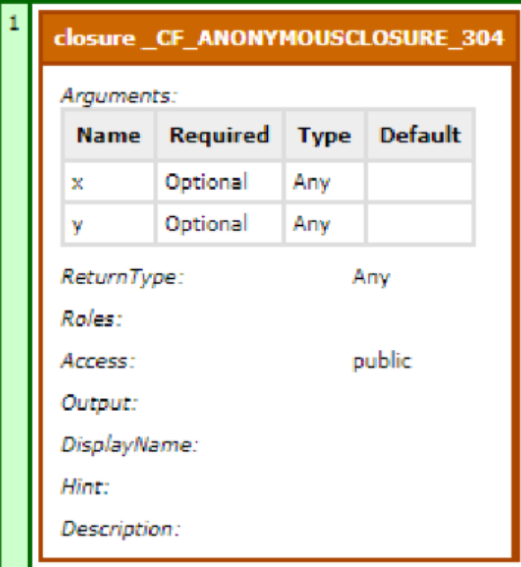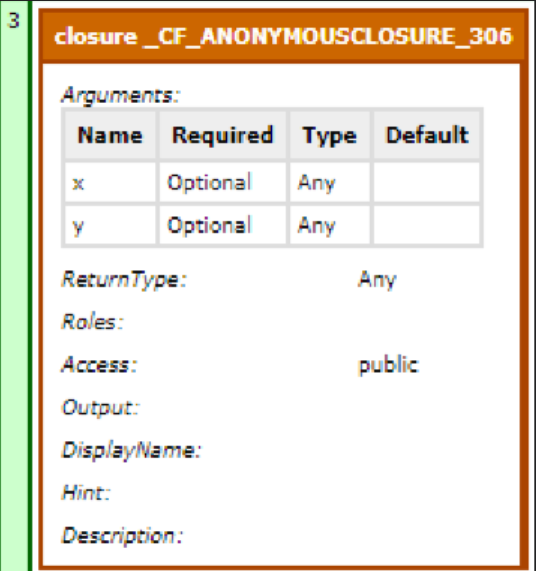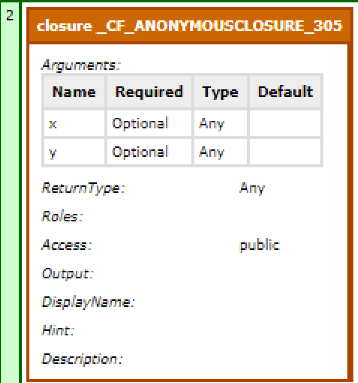Also, you can use the following snippet to see how array values can be used as function arguments:

```<cfscript>
array2 = [(arg1) => { return arg1+1 }, (arg1) => { return arg1 }]
writedump(array2)
writeDump(array2(3))
writeDump(array2())
array3 = [(String arg1=NULL) => { return arg1 }, (String arg1=2) => { return arg1 }]
writedump(array3)
writeDump(array3())
</cfscript>```

## Object literals

You can return an object literal using the arrow operator. For example,

```<cfscript>
// object literals
myfunction=()=>{return value= 'test'}
writedump(myfunction())
</cfscript>```

## Closures as arguments

```See the example below.
<cfscript>
function firstFunction1(function closure) {
if(isClosure(closure))
return closure();
return;
}

function secondFunction1(function closure,string arg1) {
return closure(arg1);
}

function thirdFunction1(string arg1, function closure, string arg2) {
return closure(arg1,arg2);
}

function fourthFunction1(function closure) {
return closure(insideClosure=() => {return 1;});
}

function fifthFunction1(function closure1, function closure2) {
return closure1(closure2);
}
</cfscript>
<cfscript>
output = firstFunction1(closure=() => {return "Hello";});
writeOutput(output);
</cfscript>

<cfscript>
output = secondFunction1(closure=(arg1) => {return arg1;},arg1="Hello");
writeOutput(output);
</cfscript>

<cfscript>
output = thirdFunction1(arg1="Hello",closure= (arg1,arg2) => { return arg1 & arg2;},arg2="World");
writeOutput(output);
</cfscript>

<cfscript>
output = fourthFunction1(closure = (insideClosure) => { return insideClosure();});
writeOutput(output);
</cfscript>

<cfscript>
output = fifthFunction1(closure1 = (closure2) => { return closure2();}, closure2 = () => { return 1;});
writeOutput(output);
</cfscript>```

## Examples- Arrow operator in Built-in Functions

In the examples below, you can see how Lambda can used to write cleaner, better code for the three most important pillars of functional programming- map, filter, and reduce.

Note:

We have used arrays for the examples.

### Map

The Map function operates on all elements of an array and produces an array of the same dimension with transformed values.

Using the arrow operator, you can implement Map function in an array as follows:

```<cfscript>
// array map using lambda
numbers=[1,4,6,9]
double=numbers.map((numbers)=>{return numbers*2})
writedump(double)
</cfscript>```

Output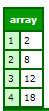### Filter

Filter, like map, operates on all elements of an array, and returns a filtered, subsetted array, depending on one or more conditions.

Using the arrow operator, you can implement Filter function in an array as follows:

```<cfscript>
// array filter using lambda
superheroes=[
{"name":"Iron Man","member":"Avengers"},
{"name":"Wonder Woman","member":"Justice League"},
{"name":"Hulk","member":"Avengers"},
{"name":"Thor","member":"Avengers"},
{"name":"Aquaman","member":"Justice League"}
];
writedump(superheroes)
filtered=superheroes.filter((superheroes)=>{
return (superheroes.member=="Avengers")
})
writedump(filtered)
</cfscript>```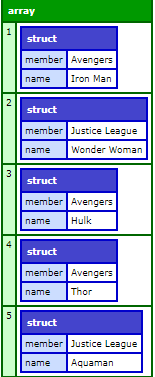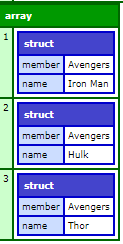### Reduce

The Reduce function “reduces” an array to a single value. Using an arrow operator, you can implement Reduce as follows:

```<cfscript>
// array reduce using lambda
numbers=[1,3,5,7,9]
sum=numbers.reduce((previous,next)=>{
return previous+next
},0)
writeOutput(sum)
</cfscript>```

### Chaining of member functions

In ColdFusion (2018 release), chaining of member functions was introduced. Using arrow operators, you can chain functions, and significantly reduce your code footprint.

For example,

```<cfscript>
// chaining of lambda functions
numbers=[1, 2, 4, 5, 6, 7, 7, 9, 11, 14, 43, 56, 89]
// find if number is even
isEven=(x)=>{return x%2==0}
// chain the functions
writedump(result)
</cfscript>```

Output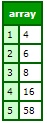## Arrow operators in ColdFusion tags

In ColdFusion (2018 release), closures in tags were introduced. For example,

```<cfset myarray=[
{name="Thomas", age="22"},
{name="Zaza", age="36"},
{name="Novak", age="136"},
{name="Marin", age="361"},
{name="Rafa", age="3"},
{name="\$bl0091@", age="-23"}
]>
<!--- define closure function --->
<cfset closure=function (e1,e2){
return compare(e1.name,e2.name);
}>
<cfset ar = arraySort(myarray,closure)>
<cfdump var="#myarray#">```

In ColdFusion (2018 release) updates, support for arrow operators has been added to tags. For example,

```<cfset print=()=>{
return "Hello World"
}>
<cfoutput>
#print()#
</cfoutput>```

In the above snippet, an empty function print is created that returns the string “Hello World”.

In ColdFusion tags, you can also include nested arrow functions and return appropriate output. For example,

```<cfset myClosure= (default)=> {
return (default) =>
{
return ()=> {
return "Hello World"
}
}}>
<cfoutput>#myClosure("testing arrow in nested levels")()()#</cfoutput>
Also,
<cfset myClosure= (default)=> {
return (default) =>
{
return (string s)=> {
return s
}
}}>
<cfoutput>#myClosure("testing arrow in nested levels")()("Hello World")#</cfoutput>```

## Using Lambdas in Named arguments

You can use arrow operators as named arguments in a function. See the examples below.

### Example 1

```<cfscript>
myArray1 = ["CF10","Zeus","CF9","Centaur"];
resultArray = ArrayFilter(array=myArray1,callback=(any arrayEntry) =>{
if(arrayEntry == "CF9" || arrayEntry == "Centaur")
return false;
return true;
});
for(var1 in resultArray) {
writeOutput(var1 & "<br>");
}
</cfscript>```

### Example 2

```<cfscript>

numArray = [10,20,30,150,400,99,100];
resultArray = ArrayFilter(array=numArray,callback=(any arrayEntry) =>{
if(arrayEntry < 100)
return false;
return true;
});
for(var1 in resultArray) {
writeOutput(var1 & "<br>");
}
</cfscript>```

### Example 3

```<cfscript>
numArray = [60,3,30,4,500,44];
</cfscript>

<cfscript>
index = ArrayFind(array=myArray1,callback=(any object1) => {
if(object1 == "Systems")
return true;
return false;

});
writeOutput("Found at " & index);
</cfscript>

<cfscript>
index = ArrayFind(myArray1,(any object1) =>{
if(object1 == "Hello")
return true;
return false;

});
writeOutput("Found at " & index);
</cfscript>```

# Closures in tags

In previous versions of ColdFusion, you could use closures in functions, but not in tags. Supporting closures in Tags would mean enabling everything that is valid within a CFScript body to be embedded inside a CFTag.

For example, the closure function in arraySort returns results as expected.

```<cfscript>
// Define an array of structs
myArray = [
{name="Thomas", age="22"},
{name="Zaza", age="36"},
{name="Novak", age="136"},
{name="Marin", age="361"},
{name="Rafa", age="03"},
{name="\$bl0091@", age="-23"}
];

// Define a closure function that sorts the names in the array of structs
callback=function (e1, e2){
return compare(e1.name, e2.name);
}

// Use the closure function
arraySort(myArray,callback);

// Display the sorted array of structs
WriteDump(myArray);
</cfscript>
```

On the other hand, the snippet below always threw an exception:

`<cfset myClosure= function() {…}>`

In the 2018 release of ColdFusion, you can use closure functions in tags. For example,

```<cfset myarray=[
{name="Thomas", age="22"},
{name="Zaza", age="36"},
{name="Novak", age="136"},
{name="Marin", age="361"},
{name="Rafa", age="3"},
{name="\$bl0091@", age="-23"}
]>
<!--- define closure function --->
<cfset closure=function (e1,e2){
return compare(e1.name,e2.name);
}>
<cfset ar = arraySort(myarray,closure)>
<cfdump var="#myarray#">
```